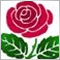# Get Values of Channel's Second Line1083

I can use ObjectGetValueByShift() to get the values of the channel's main line. But how do I get values of the second line? Is it possible at all?

Calculating using linear equation formula based on time yields incorrect results due to non-linear time change in the chart.17213

enivid:

I can use ObjectGetValueByShift() to get the values of the channel's main line. But how do I get values of the second line? Is it possible at all?

Calculating using linear equation formula based on time yields incorrect results due to non-linear time change in the chart.

Look up how the channel is drawn in the TA section of this site, from that you should be able to work it out.1083

Sorry, but that fails to provide any useful information on my inquiry.17213

enivid:
Sorry, but that fails to provide any useful information on my inquiry.

Really ?  what did you expect ?  code ?

"Linear Regression Channel consists of two parallel lines, equidistant up and down from the line of linear regression trend. The distance between frame of the channel and regression line equals to the value of maximum close price deviation from the regression line.108317213

enivid:

So you mean an Equidistant Channel ?

 OBJ_CHANNEL 5 Channel. Uses 3 coordinates.

It has 3 coordinates . . .   draw one on your chart manually,  see what the 3rd coordinate is used for.1083

Thanks but I know what the 3rd coordinate is used for. It helps little in finding out the value (price) of the second line at some given shift.

It could be calculated for cases when the 3rd coordinate is located at current bar or earlier. But it seems impossible for cases when the third coordinate is placed somewhere in the future part of the chart.17213

enivid:

Thanks but I know what the 3rd coordinate is used for. It helps little in finding out the value (price) of the second line at some given shift.

It could be calculated for cases when the 3rd coordinate is located at current bar or earlier. But it seems impossible for cases when the third coordinate is placed somewhere in the future part of the chart.

The lines are parallel,  so the price difference between the lines is constant.  Get the price difference between the 3rd coordinate and the other coordinate that is at the same time and you have the price offset between the lines . . . .   isn't that what you need ?  add this offset to the value of the ObjectGetValueByShif() and you have the value of the 2nd line at a specific bar number.  Job done.

All you needed to do was draw a channel manually on a chart and investigate it's "properties",  where the coordinates are, how they relate to each other.  It's pretty simple.1083

I wish it would be that simple. We can get the price offset between two lines only if the time of the 3rd coordinate is the same as the time of 1st or 2nd coordinates. We could even calculate the offset if the 3rd coordinate has bar shift >= 0, but I cannot find a way to get it when this shift is negative coordinate is placed in future and doesn't match time of 1st/2nd coordinate.17213

enivid:
I wish it would be that simple. We can get the price offset between two lines only if the time of the 3rd coordinate is the same as the time of 1st or 2nd coordinates. We could even calculate the offset if the 3rd coordinate has bar shift >= 0, but I cannot find a way to get it when this shift is negative coordinate is placed in future and doesn't match time of 1st/2nd coordinate.
OK,  I see . . .  why can't you extrapolate the price for the 1st/2nd coordinate to a time the same as the 3rd coordinate to get the offset ?1083

We cannot use time as x in f(x) = kx + b calculation because charts are non-linear in respect to time. For example, daily charts have weekends and holidays omitted, so the neighboring bars could be 1 day apart or 3 days apart.